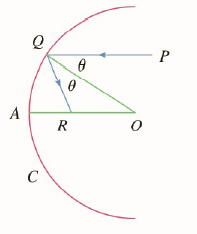Chapter 2.P, Problem 19P

Chapter
Section
Textbook Problem

# Suppose that we replace the parabolic mirror of problem 18 by a spherical mirror. Although the mirror has no focus, we can show the existence of an approximate focus. In the figure, C is a semicircle with center O. A ray of light coming in toward the mirror parallel to the axis along the line PQ will be reflected to the point R on the axis so that ∠ P Q O = ∠ O Q R (the angle of incidence is equal to the angle of reflection). What happens to the point R as P is taken closer and closer to the axis?To determine

To check:

Effect on point R as P is taken closer and closer to the axis.

Explanation

1) Concept:

Here we use Laws of Cosines to simplify given examples.

2) Formula:

Laws of cosine: Let a, b, c  be sides of triangle and A, B, C are corresponding opposite angles, then a2=b2+c2-2bc cosA.

3) Calculations:

From figure, OQR=OQP

Therefore QOR  is isosceles.

Hence, QR=RO=x

By using Laws of cosines,

x2=x2+r2-2rx co

### Still sussing out bartleby?

Check out a sample textbook solution.

See a sample solution

#### The Solution to Your Study Problems

Bartleby provides explanations to thousands of textbook problems written by our experts, many with advanced degrees!

Get Started